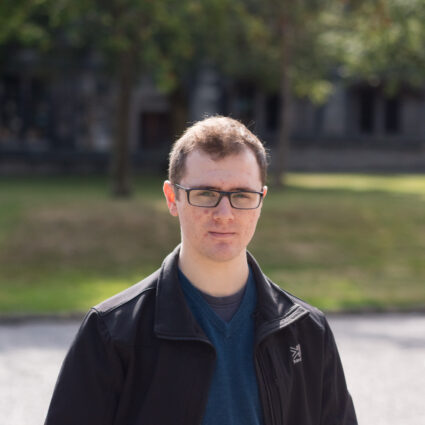# Cameron Wilson

Project Title: Hasse principle for random varieties over global fields

A Diophantine equation is a multivariable polynomial with integer coefficients and the desired solutions have only integer coordinates. A famous example is x^n + y^n=z^n, which was the focus of Fermat’s Last Theorem (proven by Andrew Wiles in the 1990s). These equations have been studied since 200AD but there are still many open questions regarding them.

Hilbert’s 10th problem (1900) was to find a finite time algorithm that could find integer solutions to any Diophantine equation. This was proven to be impossible for some equations in 1970 by Matiyasevich. Despite this, mathematicians still believe that a finite algorithm should still exist for randomly chosen equations (loosely meaning that any counter examples are very rare).

The Hasse principle is a candidate algorithm that has already been proven to work for special types of equations using algebraic and geometric ideas. Colliot-Thélène proved that this algorithm will work for the majority of surfaces on the condition that the Schinzel conjecture – which regards prime values of integer polynomials – is true. In 2020 Sofos and Skorobogatov verified Schinzel for random polynomials of any degree. By combining techniques from geometry, algebraic number theory and analytic number theory this project aims at generalising and combining these results to prove the Hasse principle for a variety of equations.Awarded: Carnegie PhD Scholarship

Field: Mathematics and Statistics

University: University of Glasgow